## IONIC EQUILIBRIUMThe ionic equilibrium established between the unionized molecules and the ions in the solution of weak electrolytes is called ionic equilibrium.
e.g.,   CH3COOH   ⇄ CH3COO + H

Electrolytes
Chemical substances which can conduct electricity in their aqueous stare or in the molten state are called electrolytes. The conduction of current through the electrolyte is due to the movement of ions. There are two types of electrolytes:

• Strong electrolytes
• Weak electrolytes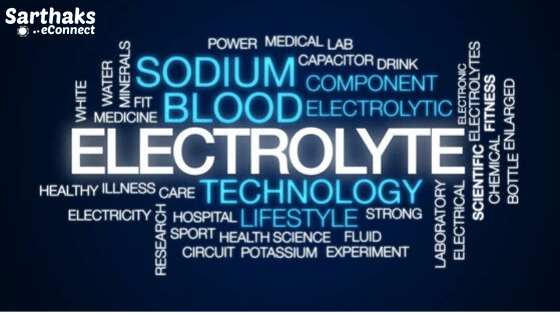1. Strong electrolytes: Electrolytes which dissociate almost completely into constituent ions in aqueous solution are known as strong electrolytes. e.g.. all salts (except HgCl, CdBr )’ mineral acids like HCl, H2SO4, HNO3etc.. and bases like NaOH. KOH. etc.
2. Weak electrolytes: Electrolytes which dissociate to a lesser extent in aqueous solution are called weak electrolyte. All organic acids (except sulphonic acids), and bases like NH3,NH4OH, amines,H3BO3, HCN, etc.

### Degree of Ionisation or Degree of Dissociation in Ionic Equilibrium (α)

It is the fraction of the total number of molecules which ionize (dissociate) into constituent ions.

α =$$\frac{ number \,of \, molecules \,ionized \,or \,dissociated}{total \, number \,of \,molecules \,taken}$$
For strong electrolytes,
α = 1
For weak electrolytes
α < 1

Values of the degree of dissociation depend upon the following factor:

1. nature of solute
2.  nature of solvent
3.  concentration
4. temperature

#### Ostwald’s Dilution Law In ionic equilibrium:

According to Ostwald. the degree of dissociation of weak electrolyte is inversely proportional to the square root of the molar concentration of the solution.

K =$$\frac{Cα^2}{{1-α}}$$

If α is very small 1-α ≈1 ⇒ K= $${C}\times{a ^2}$$

or,

α=$$\sqrt{\frac{K}{C}}$$ ⇒α∝$${\frac{1}{\sqrt{C}}}$$

Here, K is disspciation constant and C is molar concentartion of the solution.

## Acids and bases in Ionic Equilibrium:

• Earlier definitions of acids and bases were given by Robert Boyle, who classified them on the basis of their properties. According to him, acids are the substance which has a sour taste, turns blue litmus red, liberate hydrogen with metals & conduct electricity in aqueous solution and
neutralize bases.
• Bases are the substance which has a bitter taste, turns red litmus blue, soapy to touch, conduct electricity in aqueous solution and neutralise acids.

### Arrhenius Concept of Acids and Bases:

Acid is a chemical substance which dissociates in aqueous solution to give hydrogen ions (H+ ) or hydronium ions (H3O+ ).
Base is a chemical substance which dissociates in aqueous solution to give hydroxyl ions (OH- ).
Arrhenius theory fails to explain the acidic and basic behavior in non-aqueous solutions. It cannot explain the acidic character of A1Cl3. BF3 and basic character of NH3 , PH3 & free H+ and OH ion do not exists in water etc.

#### Bronsted Concept of Acids and Bases:

Acid is a chemical substance that can donate a proton (H+) to some other substance and a base is a chemical substance that can accept a proton from other substance. Thus, an acid is a proton donor (protongenic) and a base is proton acceptor (protopbilic).

NH3   +  H2O   ⇄    NH4+ + OH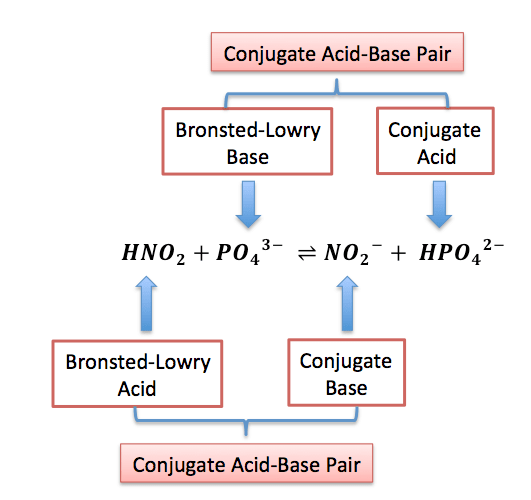• A strong acid has weak conjugate base and weak acid has a strong conjugate base.Strong base has weak conjugate acid and weak base has strong conjugate acid.
• HClO is the strongest while HCN is the weakest hydracid known.
• CsOH is the strongest base known.

Amphoteric or amphiprotic substance or ampholytes are the substance which acts as an acid as well as a base, e.g. water acts as an acid with NH3 and a base with acetic acid.The order of acidic strength of some acids is:
HClO4 > HI> HBr > H2SO4 > HC1> HNO3

• Greater the Kα value of an acid (or lesser the pKα), stronger is the acid. Similarly. greater the Kb (or lesser the pKb ) of a base. stronger is the base.

$$\frac{a_1}{a_2}=\sqrt{\frac{K_{a1}}{K_{a2}}}$$= $$\frac{strength \,of \,acid [HA_1]}{strength \,of \,acid [HA_2]}$$

##### Leveling Effect in Ionic Equilibrium:
• The acids like HClO4  H2SO4 , HNO3, etc. react with water almost completely to form H3O+ ions. Therefore, all the strong acids in aqueous solutions appear equally strong and their relative strengths in aqueous solution cannot be compared. Since H3O+ is the strongest acid in water.
• The strength of above acids come down to the level of H3O+ strength in water. Similarly.strong bases like NaOH. KOH. Ba(OH)2 come down to the strength of OH ion in the water this is Leveling effect.

Bronsted Concept fails to explain acidic nature of BF3 and AlCl

## Lewis Concept of Acids and Bases:

Lewis acid is a chemical substance which can accept a pair of electrons,  e.g.,

1.  Molecules with an incomplete octet of a central atom-like AlCl3 , BeCl2, MgCl2, etc.
2.  Simple cations like Ag+, Na+ , etc.
3. Molecules in which the central atom has vacant d-orbital, e.g., SF4, SnC14, PF3, etc

### Limitations of Lewis Concept:

• It does not explain the behavior of protonic acids such as HCl, H2SO4 , HNO3 etc
•  It does not predict the magnitude of the relative strength of acids and bases.

All Bronsted-Lowry’s acids are Lewis acids while acids need not be Bronsted-Lowry’s acids.

## The Ionization Constant of Water In Ionic Equilibrium:

Ionic product is the product of the concentration of hydronium ions and hydroxyl ion in pure water, which
remains constant at a particular temperature. It is symbolized by K. At 298 K, ionic product of water (Kw) is given as K : = [H3O+ ] [OH ] = 1 x 10-14 mol2L-2 .
The value of Kw increases with increase in temperature

We can distinguish acidic, neutral and basic aqueous solutions by the relative value of the H3O+ and OH- concentrations.

Acidic: (H30+)  >  (OH)

Neutral: (H30+)  =  (OH)

Basic:   (H30+)  <    (OH)

## The pH Scale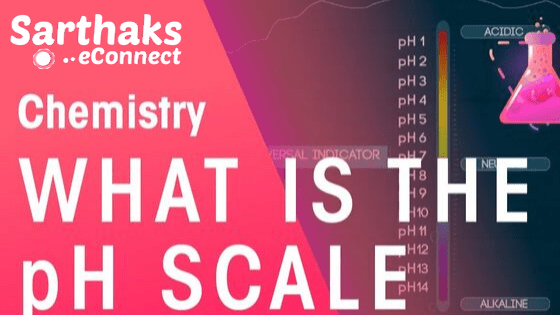pH is defined as the negative logarithm of hydrogen ion concentration.

pH = -log[H+]    and   [H+] = 10-pH

Total [H+ ] or [OH- ] in a mixture of two strong acids or bases = $$\frac{\sum NV}{\sum V}$$
Similarly, negative logarithm of hydroxyl ion concentration is pOH.

pOH= -log[H+]

As we know Kw = [H+] [OH- ] =  $${1}\times{10 ^-14}$$  (at 298 K)

-logKw = -log[H+]  –  log[OH- ]  =  14

pKw= pH + pOH = 14      (at 298 K)

• pH value of an acid having H+ concentration less than 10-7, is always in between 6 and 7. For 10-8 N HCl solution. it is 6.958.Similarly for 10-8 NaOH solution, the pH is 7.04 (because basic solutions always have pH 77.)
• pH of solution is accurately measured by pH meter or emf method or roughly by pH paper or indicator paper

PH can be zero in 1 N HCl solution or it can be negative for more. concentrated solution like 2N, 3N, lON, etc,

### Gastric juice = 1 — 3

Vinegar =2.4 — 3.4

Tears = 7.4

Human Urine= 4.8— 8.4

Blood Plasma= 7.3— 7.4

Boil Water = 6.56

## Dissociation Constant Of Weak Acid and Weak Base In Ionic Equilibrium:

Let us consider the dissociation of a weak acid (HA) as:

 HA ⇄ H + A– Initially C 0 0 At equilibrium C(1-α) Cα Cα

∴ Dissociation constant of acid

Kα = $$\frac{[{H^+}]{[A^-}]}{[HA]}$$ = $$\frac{Cα^2}{{1-α}}$$

Similarly, for the dissociation of a weak base BOH as

BOH       ⇄    B+   +   OH

∴ Dissociation constant of base

Kb= $$\frac{[{B^+}]{[OH^-}]}{[BOH]}$$ = $$\frac{Cα^2}{{1-α}}$$

Dissociation constant of polyprotic acids and bases . for Tribasic acid,

H3PO4 ⇄ H+     +    H2PO4               (Kα1)

H2PO4 ⇄ H+  +       H2PO42-             (Kα2)

HPO42-  ⇄ H+   +  PO43-                     (Kα3)

The overall Dissociation constant (k) is :

K = $${K_1}\times{K_2}\times{K_3}$$

Where. k1 > k2 > kIn ionic equilibrium

pH of H2PO4- in aqueou medium= $$\frac{pK{a_1} +pK{a_2}}{2}$$

pH of  HPO42- in aqueou medium= $$\frac{pK{a_2} +pK{a_3}}{2}$$

Similarly for dibasic acid, H2C03, pH=$$\frac{pK{a_1} +pK{a_2}}{2}$$

Similarly in case of conjugate acid —base pair,

Kw=$${K_a}\times{K _b}$$ by taking negative logorithm bot sides of eqn , then pK value of conjugate acid —base pair are related other by eqn:

pKa + pKb  =  pKw = 14 (at 298 K)

## Solubility Product in Ionic Equlibrium:

It is defied as the suppression of the dissociation of a weak electrolyte by the addition of a strong electrolyte having some common ion,e.g degree of dissociation of ammonium hydroxide decreses in the presence of ammonium chloride.

NH4OH  ⇄   NH+4  +  OH

NH4Cl → NH4+   +  Cl–                  (NH4= Common ion)

According to Le-Chatelier principle, because of the presence of common ion. degree of dissociation of NH4OH  decreases.

### Common ion effect is used in:

• Purification of common salt
•  Salting out of soap
• Qualitative analysis, II group radicals are precipitated out in the presence of HCI which suppresses the S2- ion concentration, which is just sufficient to precipitate only II group radicals.
• Similarly, in group III, NH4OH is added in presence ofNH4Cl to avoid the precipitation of V group radicals.

#### Isohydric Solutions in Ionic Equlibrium:

If the concentration of the common ions in the solution of two electrolytes, e.g., OH ion concentration in Ca(OH)2 and Ba(OH)2 solutions, is same then on mixing them there is no change in the degree of dissociation of either of the electrolytes. Such a solution is called isohydric solutions In ionic equilibrium.

## Salts:• These are the product of a reaction between an acid and a base.
• This reaction is called a neutralisation reaction.

### Types of Salts:

1.  Normal salts: These are obtained by complete neutralisation of an acid with a base, e.g., NaCI, K2SO4 , etc.
2.  Acidic salts: These are formed by incomplete neutralisation of polybasic acids. e.g., NaHCO3 , Na2HPO4 etc.
3.  Basic salts: These are formed by incomplete neutralization of polyacidic base, e.g., Mg(OH)Cl, Bi(OH)2 Cl, etc.
4. Double salts: These are formed by the combination of two simple salts and exist only in solid-state, e.g., Mohr salt or ferrous ammonium sulphate (FeSO4.(NH4)2 SO4 .6H2O], alum, etc.
5. Complex salts: These are formed by the combination of simple salts or molecular compounds. These are stable in solid-state as well as in solutions.
The properties of their solutions are different from the properties of substances from which they have been constituted.
6. Mixed salts: These salts furnish more than one cation or more than one anion when dissolved in water, e.g., Ca(OCl)Cl, NaKSO4 , etc.

#### Salt Hydrolysis in Ionic Equlibrium:

Salts are strong electrolytes and on dissolution in water split up into ions which react with H+ or OH- ions furnished by water yielding an acidic or basic solution. The process is known as salt hydrolysis.

 Salt of Example Kh(hydrolysis constant) h (degree of hydrolysis) pH of solution Weak acid and strong base CH3COONa $$\frac{K_w}{{K_a}}$$ $$\sqrt{\frac{K_w}{{K_a}\times{C}}}$$ 1/2pKw +1/2pKa+1/2log C Strong acid and weak base NH4Cl $$\frac{K_w}{{K_b}}$$ $$\sqrt{\frac{K_w}{{K_b}\times{C}}}$$ 1/2pKw-1/2pKa – 1/2log C Weak acid and weak base CH3COONH4 $$\frac{K_w}{{K_a}\times{K_b}}$$ $$\sqrt{\frac{K_w}{{K_a}\times{K_b}}}$$ 1/2pKw+1/2pKa – 1/2log C Strong acid and strong base NaCl does not undergo hydrolysis
•  An aqueos solution of a salt of strong acid and strong base is neutral.
• An aqueous solution of a salt of a weak acid and a strong base is alkaline due to anionic hydrolysis, and an aqueous solution of a salt of strong acid and a weak base is acidic due to cationic hydrolysis with dilution degree of hydrolysis increases
• Hydrolysis is a reverse process of neutralisation.

## Buffer Solution:

A solution which resists the change in its pH value by addition of a small amount of acid or a base is called buffer solution In ionic equilibrium.

Acidic buffer:  They have pH value < 7, e.g., CH3COOH /CH3COONa, bone acid/borax.
Basic buffer:   They have pH value> 7 e.g., NH4OH /NH4Cl

Buffer system present in the blood is  H2CO3  + NaHCO3 .

### Henderson-Hesselbalch Equation:

The equation used to calculate the pH of a buffer solution.

for acidic buffer: pH = pKa +log$$\frac{[salt]}{[acid]}$$

for basic buffer: pOH = pKa +log$$\frac{[salt]}{[base]}$$

pH = 14- pOH

• Here, pKa = -logKa , pKb = -logKb and Ka and Kb are dissociation constants of acid and base.
[salt), [acid] and [base] represent molar concentrations of salt, acid, and base respectively.
• If the addition of a strong acid or base changes the pH of a buffer by/unit, the buffer solution is assumed to destroyed, i.e     New  pH = pKa ± 1
• This means the ratio,

$$\frac{[salt]}{[acid]}$$ or $$\frac{[salt]}{[base]}$$ = 10 or $$\frac{1}{10}$$

#### Buffer Capacity:

It is defined as the number of moles of acid or base added in 1 L of solution Lochange the pH by unit Φ

Φ = $$\frac{no\,of \,moles \,of\, acids \,or \,base \,added \,to \,1 L \,of \,buffer}{change \,in \,pH}$$

## Solubility Product:

It is defined as the product of the concentrations of the ions of the salt in its saturated solution at a given temperature raised to the power of the ions produced by the dissociation of one mole of the salt. It is denoted by Ksp

Consider the dissociation of an electrolyte   AxBy   ⇄  x Ay+   +   yBx-

ksp=$${[A^{Y^+}]^x}\times{[B^{x^-}]^y}$$

### Application of Solubility:

1. The concept Product of Ksp helps in predicting the formation of a precipitate. In general if
• Ionic product <   Ksp , no ppt. is formed.
• Ionic product   >  Ksp , ppt. is formed.
• Ionic product   =  Ksp , the reaction is at equilibrium

2. In predicting the solubility of a sparingly soluble salt

AxBy   ⇄    x Ay+    +      yBx-

smol/L             xs                  ys

ksp =  $${x^x}\times{y ^y}\times{s^{x+y}}$$

knowing the values of K , x and y, the solubility of the salt can be Computed:

For salt of the type AB :    ksp = s2  or   s = ksp

For salt of the type AB2:   ksp =4s3  or  s= $$(\frac{K_{s_p}}{4})^{\frac{1}{3}}$$

For salt of the type AB3:  ksp = 27s4 or  s= $$(\frac{K_{s_p}}{27})^{\frac{1}{4}}$$

K of AgI is lower than t.hat of AgCl. So the former gets precipitated in preference to later.

## Distinction Between Solubility Product and Ionic Product In Equilibrium:

 Solubility Product Ionic Product It is the product of the ionic concentration in the saturated solution The Ionic Product is the ionic concentration at any concentration in the solution. It is applicable only to the saturated solution They occurs in any solution.

## Acid-Base Indicator: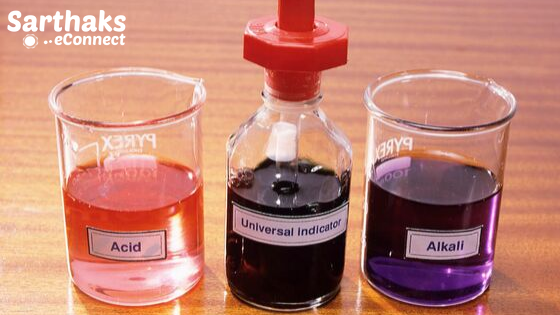• An acid-base indicator is a substance which possesses one colour in acid solution and an altogether different colour in alkaline medium In ionic equilibrium.
• Thes substance which shows colour change with change in pH. The point where the indicator shows a sudden change in colour during the titration the endpoint.
• End point is the point at which we observe the reaction to be complete.

### In Ionic Equilibrium Theory of indicators:

• Ostwald theory and
• Quinonoid theory

## Titration Curves and Indicator Used in Ionic Equilibrium: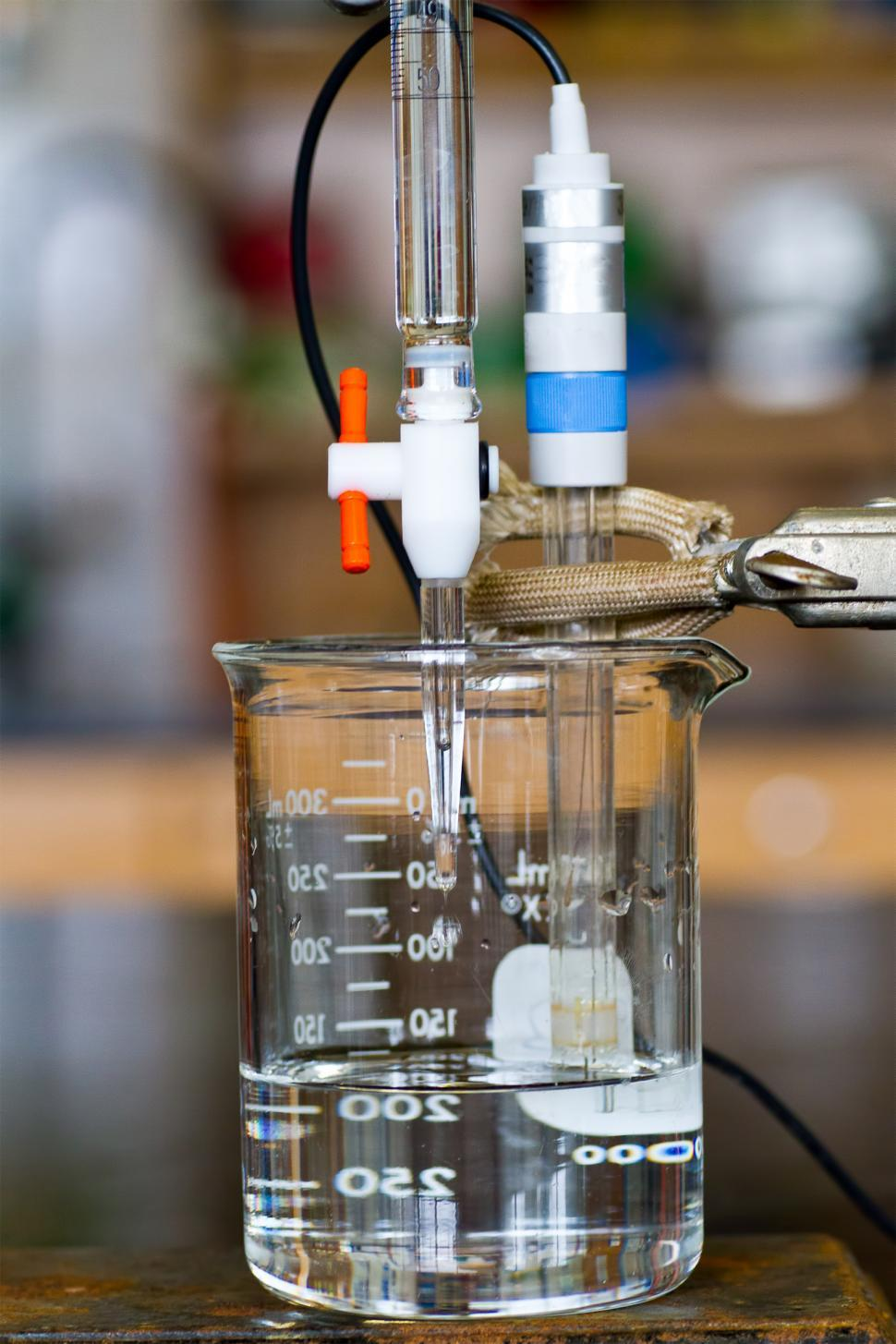1. Titration curve for the neutralisation of strong acid vs strong base: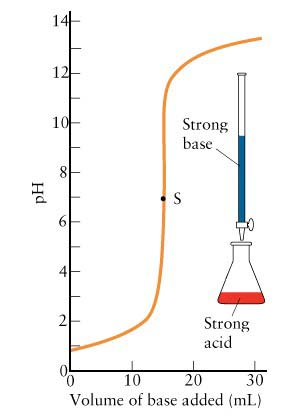In ionic equilibrium pH curve of a strong acid (say HCl) and strong base (say NaOH) is vertical over almost the pH range 4-10. So. the indicators phenolphthalein (pH range 8.3 to 10.5). methyl red (pH range 4.4-6.5 and methyl orange (pH range 3.2-4.5) are suitable for such a titration.

2.  Titration curve for the neutralisation of strong acid vs weak base: pH curve of a strong acid (say HCl or H2SO4 ” or HNO3 ) with a weak base (say NH4OH) is vertical over the pH range of 4 to 7. So the indicators methyl red and methyl orange are suitable for such a titration.

3.Titration curve for the neutralisation of weak acid vs strong base:pH curve of a weak acid (say CH3COOH or oxalic acid) and strong base (say NaOH) is vertical over the approximate pH range 7 to 11. So phenolphthalein is a suitable indicator for such a titration.

4.Titration curve for the neutralisation of weak acid vs weak base: pH curve of a weak acid and weak base indicates that there is no vertical part and hence, no suitable indicator can be used for such a titration.

## Important Question & Answers in ionic Equilibrium:

1. Calculate pH of a solution of a mixture of 10 mL 0.1 M HCl and 90 mL 0.2 M HBr

ANS: Both HCl and HBr are strong acids and therefore we can assume complete dissociation

⇒   millimoles of H from HCl = 10 x 0.1 = 1
+ millimoles of H+ from HBr = 90 x 0.2 = 18
+ Total moles of H in solution = 1 + 18 = 19
Total volume of solution = 10 + 90 = 100 mL

[H+] =  $$\frac{19}{100}$$ = 0.19M

pH = 0.72

2. The dissociation constant of CH3COOH is 1.8 x 10 . The hydrolysis constant for 0.1 M sodium
acetate is:

a. 5.56 x 104                     b. 5.56 x 10-10
c. 1.8 x 10-5                      d. 1.8 x 109

ANSHence the correct option is (b) 5.56 x 10-10

3. pH of 2 M NaCl will be:

a)  3                                   b) 6.5

c) 7                                    d) 10

ANSHence the correct option is (c) 7

4. The pH of 0.1 M solution of NaHCO3 is (Given : pKa (H2CO3 ) = 6.38 and pKa2 (HCO3 ) = 10.32)

a)  8.35                              b)  6.5

c)  4.3                                 d) 3.94

ANSHence the correct option is (a)  8.3